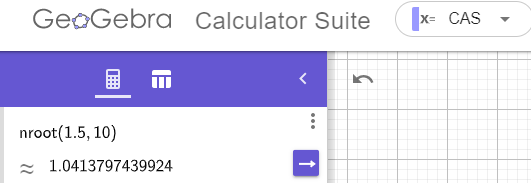# Why Geogebra cas won't solve my simple equation numerically

parthenocissus shared this question 2 years ago

Hi,

I tried to input a very simple equation in Geogebra: nroot(1.5,10) and use the numerical solve tool but I get a question mark as an answer. What's wrong, I can't figure it out?1

what is the equation?1

Works for me:You don't need to use Numerical Solve because there is no equation to solve - just a term to calculate.1

Well, I feel so stupid now that I realize this. :-D I'm just starting to learn high school maths and it's the first time in my life where I'm using something more as an aid than a simple physical calculator... Thank you!1

No problem. We've all been there!